• +91 9971497814
• info@interviewmaterial.com

# Circles Ex-10.2 Interview Questions Answers

### Related Subjects

Question 1 :

From a point Q, thelength of the tangent to a circle is 24 cm and the distance of Q from thecentre is 25 cm.

The radius of the circle is

(A) 7 cm                  (B) 12 cm

(C) 15 cm                (D) 24.5 cm

First, draw a perpendicular from the center Oof the triangle to a point P on the circle which is touching the tangent. Thisline will be perpendicular to the tangent of the circle.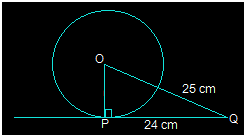So, OP is perpendicular to PQ i.e. OP PQ

From the above figure, it is also seen that OPQ is a right angled triangle.

It is given that

OQ = 25 cm and PQ = 24 cm

By using Pythagoras theorem in OPQ,

OQ2 = OP2 +PQ2

(25)= OP2+(24)2

OP2 = 625-576

OP2 = 49

OP = 7 cm

So, option A i.e. 7 cm is the radius of thegiven circle.

Question 2 :

In Fig. 10.11, if TPand TQ are the two tangents to a circle with centre O so that POQ = 110°,

then PTQ is equal to

(A) 60°                      (B) 70°

(C) 80°                      (D) 90°

From the question, it is clear that OP is theradius of the circle to the tangent PT and OQ is the radius to the tangents TQ.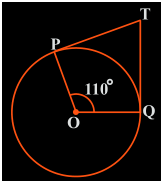So, OP PT and TQ OQ

∴∠OPT = OQT = 90°

Now, in the quadrilateral POQT, we know thatthe sum of the interior angles is 360°

So, PTQ+POQ+OPT+OQT = 360°

Now, by putting the respective values we get,

PTQ +90°+110°+90° = 360°

PTQ = 70°

So, PTQ is 70° which isoption B.

Question 3 : If tangents PA and PB from a point P to a circle with centre O are inclined to each other at angle of 80°.

then POA is equal to

(A) 50°                            (B) 60°

(C) 70°                            (D) 80°

Solution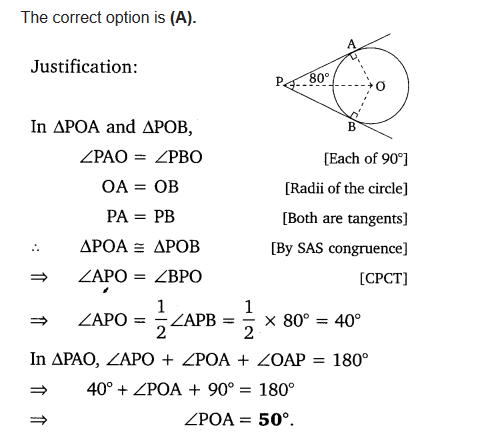Question 4 :

Prove that thetangents drawn at the ends of a diameter of a circle are parallel.

Answer 4 :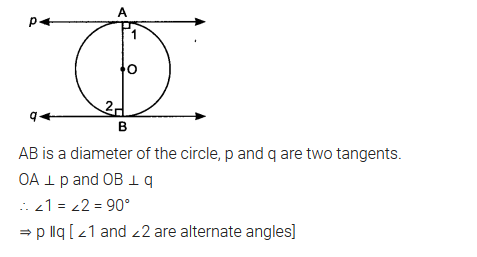Question 5 :

Prove that theperpendicular at the point of contact to the tangent to a circle passes throughthe center.

Answer 5 :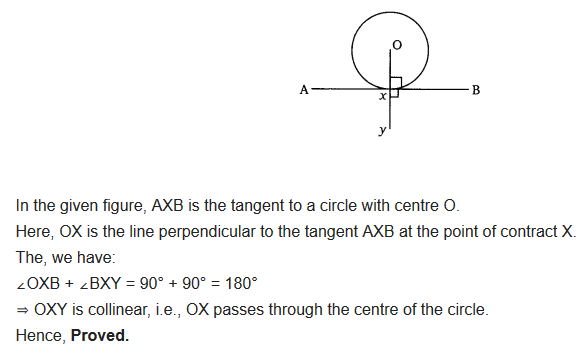Question 6 :

The length of atangent from a point A at distance 5 cm from the centre of the circle is 4 cm.Find the radius of the circle.

Answer 6 :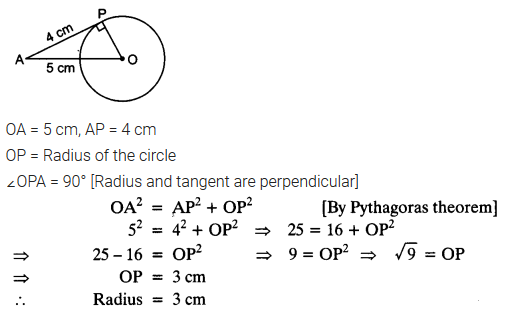Question 7 :

Two concentriccircles are of radii 5 cm and 3 cm. Find the length of the chord of the largercircle which touches the smaller circle.

Answer 7 :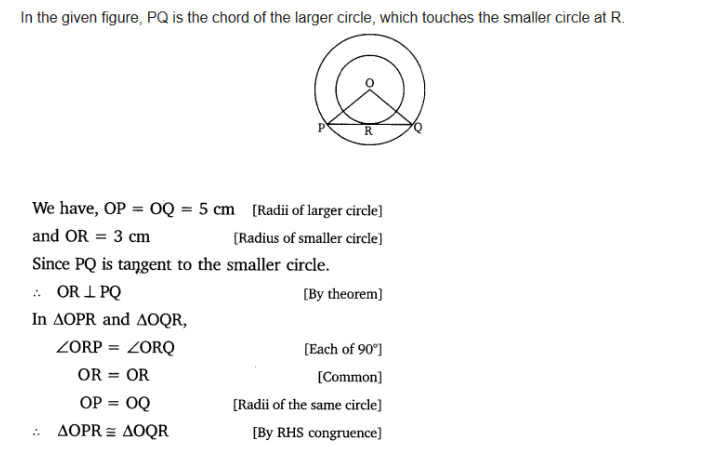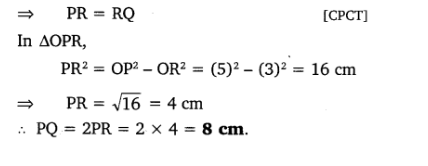Question 8 :

A quadrilateral ABCDis drawn to circumscribe a circle (see Fig. 10.12). Prove that AB + CD = AD +BC

Answer 8 :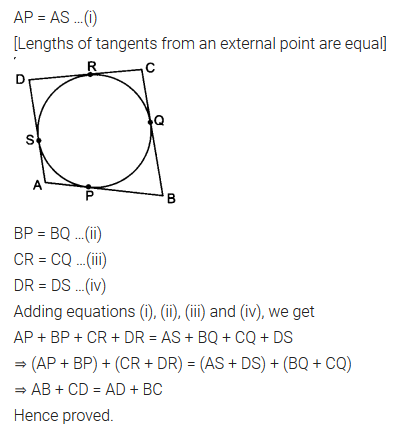Question 9 : In Fig. 10.13, XY and X′Y′ are two paralleltangents to a circle with centre O and another tangent AB with point of contactC intersecting XY at A and X′Y′ at B. Prove that AOB = 90°.

Answer 9 :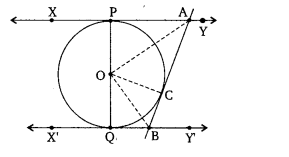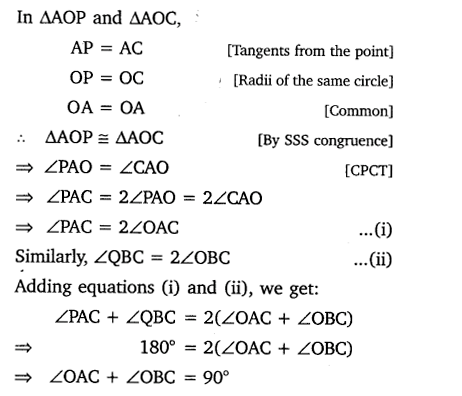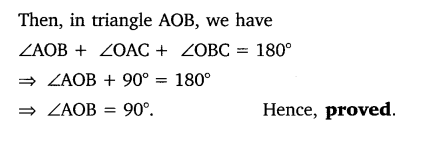Question 10 :

Prove that the anglebetween the two tangents drawn from an external point to a circle issupplementary to the angle subtended by the line-segment joining the points ofcontact at the center.

Answer 10 :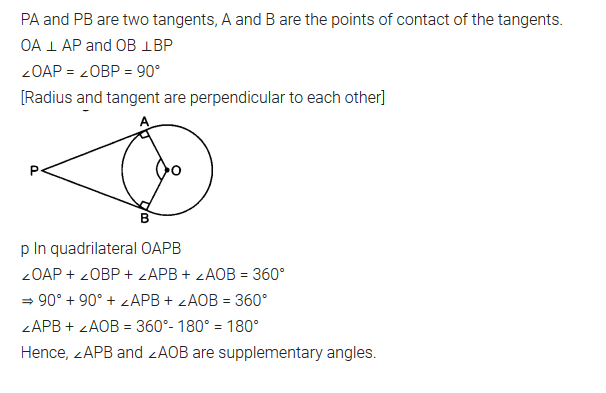Todays Deals### Circles Ex-10.2 Contributorskrishan

Name:
Email:

# Latest News# 9000 interview questions in different categories